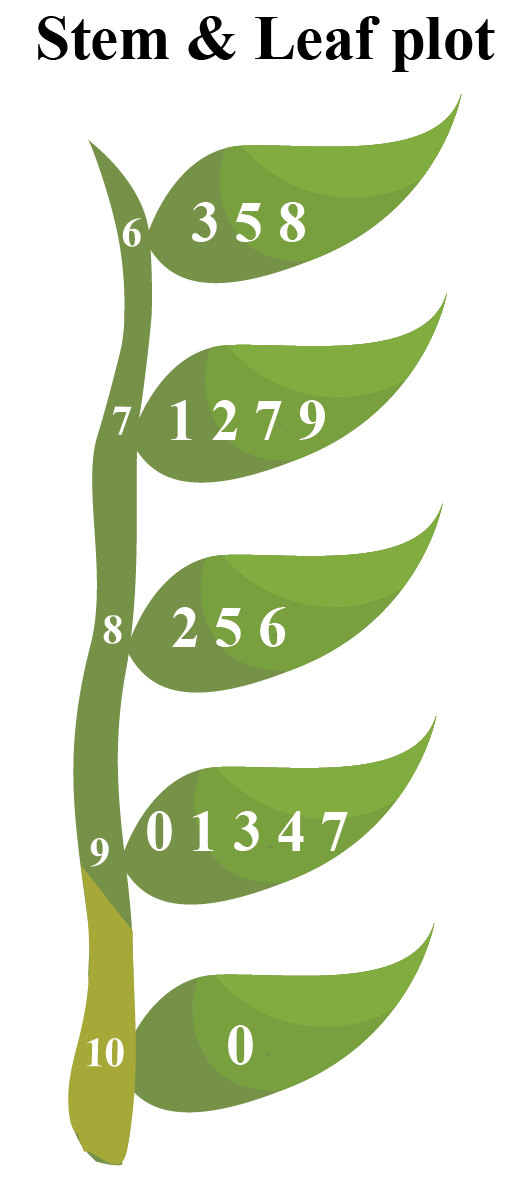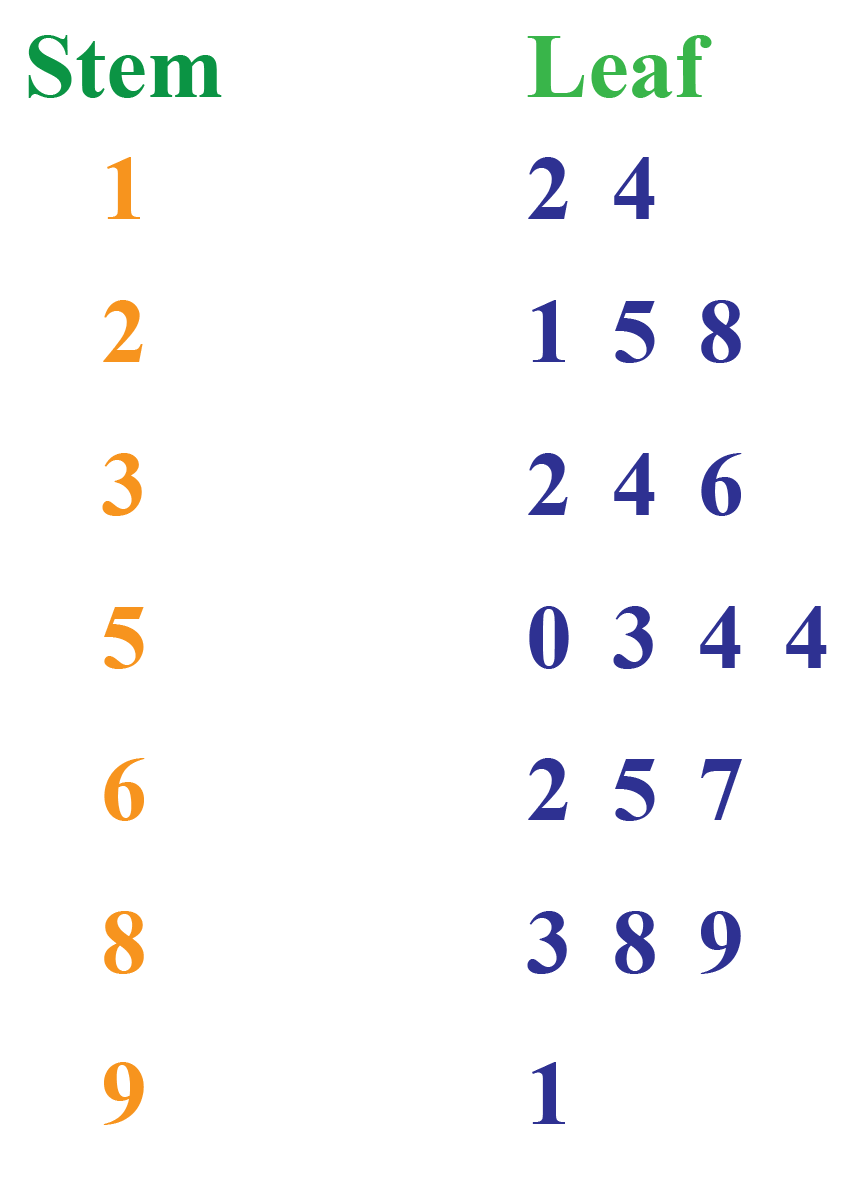# Stem and Leaf Plot

A stem-and-leaf plot or stem-and-leaf display presents the quantitative data in a graphical format, thus assisting in visualizing the shape of a distribution.

Stem and leaf plots are similar to horizontal bar graphs, except actual numbers are used instead of bars in stem and leaf plots.

In this chapter, we will learn about stem and leaf plot maker, stem and leaf plot key, and stem and leaf plot excel in the concept of stem and leaf plot. Check out the interactive simulation to generate the stem and leaf plot statistics.

Do not forget to try your hand at solving a few interesting practice questions at the end of the page for a quick revision.

## Lesson Plan

 1 What is Stem and Leaf Plot? 2 Thinking Out of the Box! 3 Important Notes on Stem and Leaf Plot 4 Solved Examples on Stem and Leaf Plot 5 Interactive Questions on Stem and Leaf Plot

## What is Stem and Leaf Plot?

A stem and leaf plot is a graph that shows numerical data arranged in order.

Each data value is broken into a stem and a leaf.

stem and leaf plot is a way of organizing data into a form that makes it easy to observe the frequency of different types of values.

### Stem and Leaf Plot Definition

It is a special table where each data value is split into a stem (the first digit or digits) and a leaf (the last digit of the data).

$$46$$ is represented as $$4$$ on the stem and $$6$$ on the leaf.

The stem values are listed one below the other in ascending order and the leaf values are listed left to right from the stem values in ascending order.

ExampleAs the stem and leaf plot definition states,

$$6$$ on the stem and $$3$$ on the leaf is read as $$63$$

$$9$$ on the stem and $$0$$ on the leaf is read as $$90$$

$$10$$ on the stem and $$0$$ on the leaf is read as $$100$$

### Stem and Leaf Plot With Decimals

We can use stem and leaf plot with decimals the same way we use the stem and leaf plot with whole numbers.

The stem and leaf plot key helps us understand how the data is organised on the stem and leaf plot with decimals.

Example

stem and leaf plot key: $$4$$ I $$2$$ is $$4.2$$

Stem Leaf
1 8
2 1  2
4 2  5
5 3   7

As per the stem and leaf plot definition and the stem and leaf plot key, the data values are $$1.8, 2.1, 2.2, 4.2, 4.5, 5.3, 5.7$$

### Stem and Leaf Plot Statistics

Consider the following stem and leaf plot worksheet which shows 5 data values.

Finding the mean, median and mode are a part of stem and leaf plot statistics.

Stem Leaf
2 0
3 2  2  5
4

The data values are already in ascending order. They are 20, 32, 32, 35 and 41

\begin{align}\text{Mean of the data} &= \text{Sum of data values}\div\text{Total number of values}\\ &= (20+32+32+ 35 +41)\div5 \\&=160\div5\\\text{Median}&=32\end{align}

Mode is the data value that frequently appears.

$\text{Mode}= 32$

Median = The middle value of the data.

$\text{Median} =32$

Thus calculating the stem and leaf plot statistics is made easy.

## Seven Steps of Constructing a Stem and Leaf Plot

Step 1: Look at the data and find the number of digits. Classify them as 2 or 3 digit numbers.

Step 2: Fix the stem and leaf plot key.

For example: $$3$$  I  $$5$$ = $$35$$ and $$15$$  I  $$2$$ is $$152$$

Step 3: Identify the first digits as stems and the last digits as leaves.

Step 4: Determine the range of the data, i.e. the lowest and the highest values among the data.

Step 5: Draw a vertical line. Place the stem on the left column and the leaf on the right column.

Step 6: List the stems in the stems column. Arrange it in the ascending order starting from the lowest at the top.

Step 7: Plot the leaves in the column against the stem from lowest to the highest horizontally.

The simulation shown below on the stem and leaf plot maker helps you construct a stem and leaf plot.

The simulation helps you generate the data.

You can observe the stem and leaf plot statistics being generated accordingly.

## How Do You Find the Stem and Leaf Plot?

The stem and leaf plot key helps us understand the data values.

The stem is on the left and the leaf is on the right.

If you combine the values of the stem and the leaves, you will get the data values.

Let us interpret the following data after studying the stem and leaf plot.

Stem Leaves
$$0$$ $$2$$  $$6$$  $$8$$
$$1$$ $$5$$ $$7$$
$$2$$ $$5$$  $$9$$

(a) What are the leaf numbers for stem $$2$$?

Observe the stem and leaf plot worksheet.

Look at the stem column on the left and locate stem $$2$$. Then find the corresponding leaves on the right.

The leaf numbers are $$5$$ and $$9$$

(b) What are the data values for stem $$2$$?

Observe the stem and leaf plot worksheet. Identify the data values.

The stem means $$20$$ and the leaf means $$5$$ and $$9$$

Combining the values of the stem and the leaves, we get the data values as $$25$$ and $$29$$

(c) What are the data values for stem $$0$$?

The numbers on the leaves against the value $$0$$ of the stem are $$2$$, $$6$$, and $$8$$

Thus, the data values are $$2$$, $$6$$, and $$8$$

(d) List the data values greater than $$15$$

From stem value $$1$$, we get the data values $$15$$ and $$17$$

From stem value $$2$$, we get the data values $$25$$ and $$29$$

Hence, the data values greater than $$15$$ are $$17$$, $$25$$, and $$29$$Think Tank
1. The stem and leaf plot can be plotted using excel sheet the same way, as we do in stem and leaf plot worksheets.
2. The stem and leaf plot excel helps in organising the data better.
3. The countIf formula is used to compute the leaves for the first row and then can be repeated for the subsequent rows in the stem and leaf plot excel.

## How Do You Split a Stem and Leaf Plot?

The split stem and leaf plot separates each stem into many stems based on its frequency.

We place the smaller leaves on the first part of the split stem and the larger leaves on the subsequent stems.

For example, let's consider a set of data that includes the scores of 8 students in their math test.

$$78$$ , $$82$$ ,$$82$$ ,$$90$$ ,$$94$$ ,$$81$$ ,$$72$$ ,$$67$$

### Stem And Leaf Plot

Stem and leaf plot
$$6$$ $$7$$
$$7$$ $$2$$    $$8$$
$$8$$ $$1$$   $$2$$  $$2$$
$$9$$ $$0$$   $$4$$

### Split Stem And Leaf Plot

Stem and leaf plot
$$6$$ $$7$$
$$7$$ $$2$$
$$7$$ $$8$$
$$8$$ $$1$$
$$8$$  $$2$$
$$8$$ $$2$$
$$9$$ $$0$$
$$9$$  $$4$$

Thus, the split stem and leaf plot is an ordinary stem and leaf plot with each instance of the stem-leaf shown separately. It represents the stem section as many entries like how it appears in the data values.Important Notes
• If we plot data using stem and leaf and place it alongside the new data, we might be able to see a correlation between both the data and the frequency distribution of data.
• Stem and leaf plotkey for three-digit numbers is represented as two digits in the stem and one digit in the leaf. For example, $$45$$ I $$6$$ = $$456$$
• Mean, median, and mode of the given data are easily calculated using stem and leaf plot.

## Solved Examples on Stem and Leaf Plot

 Example 1

Given below is the stem and leaf plot of the people who attended an election campaign from various places.

Jim is trying to read the plot, but he has many doubts.

Can you clarify them?

i) What are the data values of stem $$2$$?

ii) How many values are less than $$16$$?

Stem Leaf
$$1$$ $$4$$ $$4$$ $$6$$
$$2$$ $$3$$ $$8$$
$$3$$ $$2$$
$$4$$ $$0$$ $$1$$

Solution

i) Stem $$2$$ has $$2$$ leaves that are $$3$$ and $$8$$

Hence, the data values are $$23$$ and $$28$$

ii) Looking for the corresponding leaf value of stem $$1$$, we have $$4$$, $$4$$, and $$6$$ in the leaf column.

Thus, we find that the value less than $$16$$ is $$14$$ and it appears twice.

 $$\therefore$$ i) 23 and 28 are the data values ii) Two values are less than 16.
 Example 2

Richard is trying to read the plot given below.

His teacher has given him stem and leaf plot worksheets.

Can you help him answer the questions?i) What is the mode of the plot?

ii) What is the mean of the plot?

iii) Find the range.

Solution

i) Mode is the number that appears often in the data.

The leaf 4 occurs twice on the plot against the stem 5.

Hence, mode = 54

ii) To find the mean, we have to add up all the data values and divide the sum by the total number of values.

\begin{align}\text{mean}&=\text{Sum of all data values} \div 19 \\ &= 958 \div 19\\ &= 50.42\end{align}

iii) \begin{align}\text{Range}&= \text{the highest value - the lowest value}\\&= 91 - 12\\&= 79\end{align}

 $$\therefore$$i) Mode= $$54$$ ii) Mean = $$50.42$$ iii) Range=$$79$$
 Example 3

A store owner created the following stem and leaf plot worksheets showing the number of spectacles at each of his stores in the city.

Stem Leaf
$$1$$
$$2$$ $$4$$ $$5$$ $$7$$
$$3$$ $$2$$
$$4$$ $$2$$
$$5$$ $$3$$ $$7$$  $$8$$

i) Find which store has 20 spectacles.
ii) How many stores have more than 40 spectacles?

Solution

i) The least value in the data is $$24$$.

Therefore, we can say that no store has 20 spectacles.

ii) Looking for the corresponding leaf values of the stem 4 and above, we have 42, 53, 57, 58 which are more than 40.

 $$\therefore$$ i) No store has 20 spectacles ii) 4 stores have more than 40 spectacles

## Interactive Questions on Stem and Leaf Plot

Here are a few activities for you to practice.

## Let's Summarize

We hope you enjoyed learning about the stem and leaf plot with the simulations and practice questions. Now you will be able to easily solve problems on stem and leaf plot maker,  stem and leaf plot excel,  and stem and leaf plotkey.

At Cuemath, our team of math experts is dedicated to making learning fun for our favorite readers, the students!

Through an interactive and engaging learning-teaching-learning approach, the teachers explore all angles of a topic.

Be it worksheets, online classes, doubt sessions, or any other form of relation, it’s the logical thinking and smart learning approach that we, at Cuemath, believe in.

## 1. How do you find the mean in a stem and leaf plot?

Find all the data values corresponding to each stem and its leaves.

Add them up. Divide the sum by the total number of data.

Mean = sum of all the data values $$\div$$ total number of data values

For example,

Stem Leaf
$$1$$ $$3$$  $$6$$  $$7$$
$$2$$ $$2$$
$$3$$ $$3$$  $$9$$

The data values $$13$$,$$16$$,$$17$$,$$22$$,$$33$$ and $$39$$

Mean is

$$(13 +16+17+22+33+ 39)\\\div 6$$ = $$140 \div 6$$ = $$23.33$$

More Important Topics
Numbers
Algebra
Geometry
Measurement
Money
Data
Trigonometry
Calculus